Linear Equations

Parent topic:
Equations
•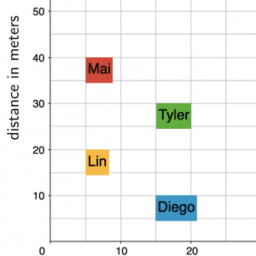Using Graphs to Compare Relationships: IM 7.2.12

Book

GeoGebra Classroom Activities

•Using Water Efficiently: IM 7.2.15

Book

GeoGebra Classroom Activities

•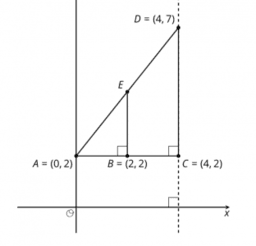Writing Equations for Lines: IM 8.2.11

Book

GeoGebra Classroom Activities

•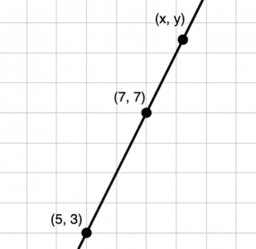Using Equations for Lines: IM 8.2.12

Book

GeoGebra Classroom Activities

•Book

GeoGebra Classroom Activities

•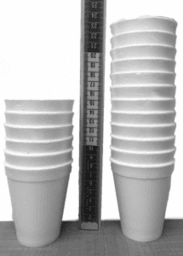Introduction to Linear Relationships: IM 8.3.5

Book

GeoGebra Classroom Activities

•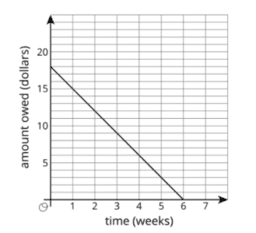Slopes Don't Have to be Positive: IM 8.3.9

Book

GeoGebra Classroom Activities

•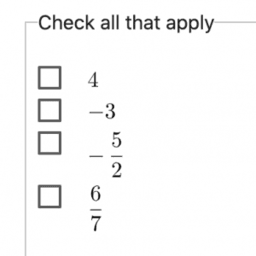Calculating Slope: IM 8.3.10

Book

GeoGebra Classroom Activities

•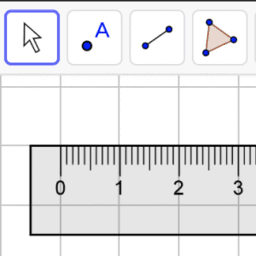Reasoning about Equations and Tape Diagrams Part 1: IM 7.6.4

Book

GeoGebra Classroom Activities

•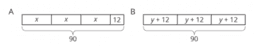Distinguishing between Two Types of Situations: IM 7.6.6

Book

GeoGebra Classroom Activities

•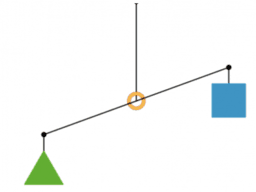Reasoning about Solving Equations (Part 1): IM 7.6.7

Book

GeoGebra Classroom Activities

•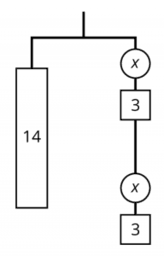Reasoning about Solving Equations (Part 2): IM 7.6.8

Book

GeoGebra Classroom Activities

•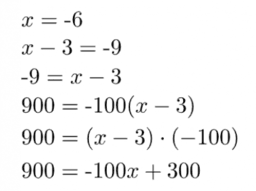Dealing with Negative Numbers: IM 7.6.9

Book

GeoGebra Classroom Activities

•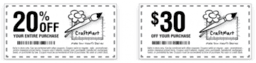Applications of Expressions: IM 7.6.23

Book

GeoGebra Classroom Activities

•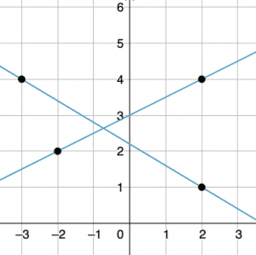Equations of All Kinds of Lines: IM 8.3.11

Book

GeoGebra Classroom Activities

•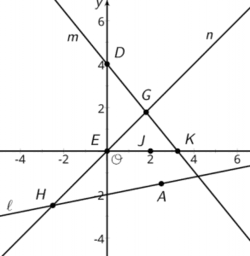More Solutions to Linear Equations: IM 8.3.13

Book

GeoGebra Classroom Activities

•More Linear Relationships: IM 8.3.6

Book

GeoGebra Classroom Activities

•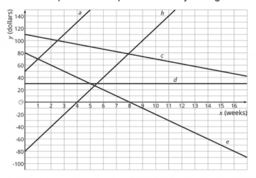Using Linear Relations to Solve Problems: IM 8.3.14

Book

GeoGebra Classroom Activities

•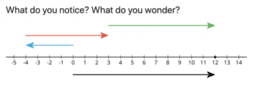Number Puzzles: IM 8.4.1

Book

GeoGebra Classroom Activities

•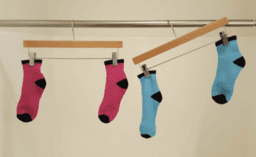Keeping the Equation Balanced: IM 8.4.2

Book

GeoGebra Classroom Activities

•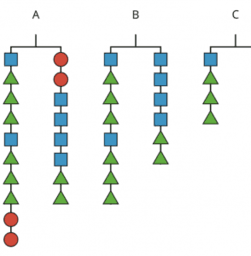Balanced Moves: IM 8.4.3

Book

GeoGebra Classroom Activities

•More Balanced Moves: IM 8.4.4

Book

GeoGebra Classroom Activities

•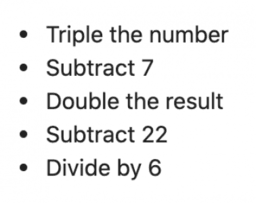Solving Any Linear Equation: IM 8.4.5

Book

GeoGebra Classroom Activities

•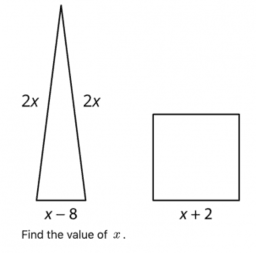Strategic Solving: IM 8.4.6

Book

GeoGebra Classroom Activities